Next: Kripke-type Semantics Up: The Formal Systems Previous: Well formed formulas

## Hilbert-type system

We now define the modal system KT5. The axiom schemata for KT5 are as follows:

(A1)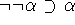(A2)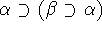(A3)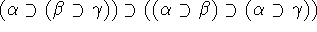(A4)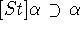(A5)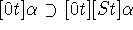(A6)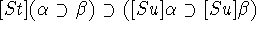, where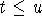(A7)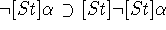We have the following two inference rules: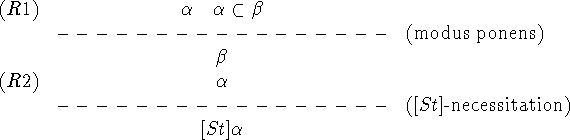We write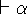if there exists a proof of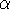. For any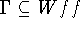we write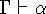if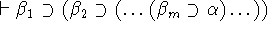for some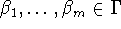.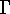is said to be consistent if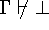.

Yasuko Kitajima
Fri Jun 20 13:39:43 PDT 1997